## Social Question# Anyone solve Will Shortz's two week challenge (see details)?

Asked by LostInParadise (29005) October 16th, 2017

You can see the challenge here It is about half way down the page under Next Week’s Challenge, which is really a two week challenge. It looked like it would be easy, but it has me stumped.

Here is how I approached it. I simplified things by turning the problem into an addition problem by moving the subtracted quantity to the other side. I also ignored the 2 digit move restriction. I just wanted to find a way of using the digits from 1 to 6 to get two numbers to add to a third.

We can eliminate the possibility of the sum being a 3 digit number. The smallest it could be is 123, which is out of range of any two digit plus one digit number.

We have ab + cd = ef. Assume no carry. a+c = e and b+d = f. a+c+e must be an even number and so must b+d+f. But that would mean the sum of the digits from 1 to 6 is even, which it is not because 1+2+3+4+5+6=21.

Suppose then that there is a carry. The only possibility is that b and d are 6 and 5 and f=1. That leaves 2, 3 and 4. The largest the sum digit e could be is 5, and 3+4=7 is already greater than 5 without the carry.

Shortz said there is no trick in the problem wording. What am I missing?

Observing members: 0Composing members: 0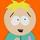Interesting puzzle. I will work on it.

filmfann (49148)“Great Answer” (1) Flag as…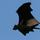I’ve only just now seen the puzzle, and I haven’t even begun to “actually” work it out, but here’s the methodology that I’ll use to start.

Given the six digits in the puzzle: 6, 5, 4, 3, 2 & 1 (irrespective of the actual numbers that they combine to create), the first part of the puzzle will be to determine the digits that go in the ones column, and that starts to narrow the choices available.

For example:
6 – 5 = 1, so that’s a possible combination
6 – 4 = 2
6 – 3 = 3 is not a possible solution, because there’s only one 3 (no more impossibles listed)
6 – 2 = 4
6 – 1 = 5
5 – 4 = 1
5 – 3 = 2
5 – 2 = 3
5 – 1 = 4
4 – 3 = 1
4 – 1 = 3
3 – 2 = 1

So that’s going to limit the choices of possible solutions to larger numbers that end in either 6, 5, 4 or 3, and smaller numbers that have only the selected digits for the selected operand. (Is that the right word? What is the word for a number that’s going to be subtracted from?)

CWOTUS (26082)“Great Answer” (1) Flag as…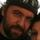@CWOTUS Substractee?

rebbel (33252)“Great Answer” (1) Flag as…In the words of Mr. Spock, your strategy reflects two dimensional thinking.

Consider, as an example, moving the 5 after the 43, as a base 5 symbol. That makes 43=23.
Also, consider moving the 2 after the 6 as a square. That makes the 6=36.
Of course, this is not the answer, but my guess is it uses this technique.

filmfann (49148)“Great Answer” (2) Flag as…You may be right, but based on other problems from Shortz, when he says there are no tricks in the statement of the problem, you can take him at his word.

The subtrahend is subtracted from the minuend. Words you are not likely to run into very often.

LostInParadise (29005)“Great Answer” (2) Flag as…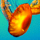I tried to brute force this with a quick program and I don’t believe there’s a straightforward solution. At least running millions of random draws for the digits 1–6 and putting them in the equation didn’t return any true answers.

So @filmfann may be on to something.

65 – (4 to the 3rd) = (1 to the 2nd) for example… and moves two digits.

displaying math here keeps getting marked up, so hopefully the above makes sense

ETA: I’d be the first to admit my program may be missing something, but it did pass several test cases

funkdaddy (17765)“Great Answer” (1) Flag as…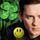@funkdaddy @filmfann I got it – Thanks to you two!
I can PM if you need it.

LuckyGuy (40060)“Great Answer” (1) Flag as…Thinking about what @filmfann said, I came up with the same answer as @funkdaddy . @LuckyGuy , did you think of something else?

LostInParadise (29005)“Great Answer” (1) Flag as…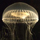An oldie but a goodie?

65 – 4³ = 1²

IM<HO superscripts denoting exponentiation involve reducing the size of the character, ergo > 2 moves.

Pinguidchance (5027)“Great Answer” (1) Flag as…That is the answer Will Shortz gave. The puzzle solver they chose for the show mentioned he had thought of the possibility of changing the 6 to a 9, which I had not thought of. It would not change my initial reasoning. Although the sum of the digits would now be even, use of 9 forces a carry, so my even/odd parity argument still holds.

LostInParadise (29005)“Great Answer” (1) Flag as…